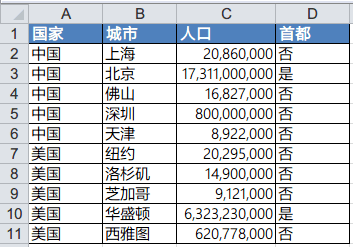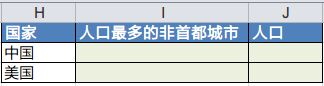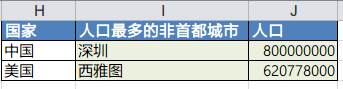# Excel数组公式的一个运用实例

``=VLOOKUP(Lookup Value,Table Range,Column Index,Match Type)``

VLOOKUP比较简单，可是有一些局限性。比如作为索引的列必须在引用的目标列的左边（Column Index不能为负），不能支持多条件的查找（当A=2，B=3时，C的值）等。在Excel中，有一个公式可以替代VLOOKUP，并且拥有更加丰富的功能，那就是INDEX MATCH数组公式。下面我们用一个实例来说明如何运用这几个公式，达到灵活查找的效果。1. 求最大值

``=MAX(Data Range)``

``=MAX(某国家非首都的人口数的集合)``

2. 查找需要的人口数集合

``=INDEX(Data Range,Row Number,Column Number)``

``=MAX(INDEX(某国家非首都的数据区域,0))``

3. 筛选某国非首都的数据区域

``=IF(Condition,Value if True,Value if False)``

``=IF(D:D="否",C:C)``

``=IF(Condition1*Condition2*Condition3...,Value if True,Value if False)``

``=IF((A:A=H2)*(D:D="否"),C:C)``

``=MAX(INDEX(IF((A:A=H2)*(D:D="否"),C:C),0))``

4. 查找对应的值

``=MATCH(Lookup Value,Data Range,Match Type)``

``=MATCH(MAX(INDEX(IF((A:A=H2)*(D:D="否"),C:C),0)),C:C,0)``

``=INDEX(B:B,MATCH(MAX(INDEX(IF((A:A=H2)*(D:D="否"),C:C),0)),C:C,0))``